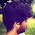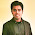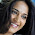## Binary Search Tree Iterator

Implement an iterator over a binary search tree (BST). Your iterator will be initialized with the root node of a BST.

Calling next() will return the next smallest number in the BST.

Note: next() and hasNext() should run in average O(1) time and uses O(h) memory, where h is the height of the tree.

## Analysis

So this problem is a little tricky if you do not familar with recursion and stack. I had an answer using recursion until recently I tried to solve this problem again by using a stack. The advantage of using a stack is making the solution pretty neat and clean. However, the disadvantage now becomes of how could we get the idea of such solution.

Firstly, let's review the basic concept of binary search tree. In short, the binary search tree is an binary tree with order, by traverse the whole tree, you will get the nodes in order. The traversal is defined how you search the tree, here in BST, we could just use the pre-order traversal. The figure below illustrates a BST and its traversal procedure.

For this specific problem, note that, the starting node for the iterator may NOT be the root node! Yes, although it is initialized from the root node, actually the starting node should be the node with smallest value (it's possible the smallest node is the root.) So what is the smallest node in the BST, it is the left-most node.

Secondly, I draw the firgure to show how the preorder traversal is done in recursion way. Specifically, in each recursion, we have 3 steps, (1) search the left node, (2)print the value, and (3) search the right node. In the figure, I show the current value and all 3 steps in blue color, this is what actually is stored in the function stack. The green arrow is our function call each time, while the organe arrow is the recursion. The red arrow and number is getting the node value.

From the figure we can see that, the whole procedue acts like the following way:

1. keep searching the left node.
2. if there is no left node OR the left node has already been visited, we do the following steps:
1. pop/print the current node
2. keep searching the right node

If we tried to simulate the function stack using a regular stack, we could see from the figure that, green arrow acts like the push to stack operation, and red arrow acts like the pop operation. The property of stack itself servers the orange arrow.

Notice that, now the top element of stack is alway the smallest node. Every time we pop the top node, at the same time we push the right child and all its left children. Because for each node, the next node in its preorder traversal will be only two cases:

1. The left-most node of its right child (if current node has right child)
2. It current node doese have right child. Then search up to its parent, until the parent node has right child. The left-most node of that right child is the next node we want.

The above two steps becomes quite easy if we are using a stack. The first step in stack is just pushing nodes into stack, while the second step could be viewed as the following steps in stack:

1. If current node has right child, we keep pushing the left child of that node (include the right child itself).
2. If there is no right child, we do nothing. The top node in the stack is what we want. Why? because once we visited the node, we pop it form the stack, so all the previous nodes are no longer existed in the stack.

In this way, we could design the iterator very easily, as the following code:

## Code (C++):

/**
* Definition for binary tree
* struct TreeNode {
* int val;
* TreeNode *left;
* TreeNode *right;
* TreeNode(int x) : val(x), left(NULL), right(NULL) {}
* };
*/
class BSTIterator {
public:
BSTIterator(TreeNode *root) {
st = stack<TreeNode*>();
push_left_node(st, root);
}
/** @return whether we have a next smallest number */
bool hasNext() {
return !st.empty();
}
/** @return the next smallest number */
int next() {
TreeNode* tmp = st.top();
st.pop();
push_left_node(st, tmp->right);
return tmp->val;
}
private:
stack<TreeNode*> st;
void push_left_node(stack<TreeNode*>& st, TreeNode* root){
while (root != NULL){
st.push(root);
root = root->left;
}
}
};
/**
* Your BSTIterator will be called like this:
* BSTIterator i = BSTIterator(root);
* while (i.hasNext()) cout << i.next();
*/

## Code (Python):

# Definition for a binary tree node
# class TreeNode(object):
# def __init__(self, x):
# self.val = x
# self.left = None
# self.right = None
class BSTIterator(object):
def __init__(self, root):
"""
:type root: TreeNode
"""
self.st = []
self.push_left(root)
def hasNext(self):
"""
:rtype: bool
"""
return len(self.st)!=0
def next(self):
"""
:rtype: int
"""
tmp = self.st.pop(0)
self.push_left(tmp.right)
return tmp.val
def push_left(self, root):
while root:
self.st.insert(0, root)
root = root.left
# Your BSTIterator will be called like this:
# i, v = BSTIterator(root), []
# while i.hasNext(): v.append(i.next())

1.Your C++ code will give a problem when the question asked on some OJ does include the variables. Rest is really good code.

2.Your iteration takes log (n) foreach step in iteration. Over all it takes n log n to iterate over all elements.

Instead if u can sacrifice on using a stack, it's additional O(log n) space (assuming balanced tree) but each call to iterator is constant operation O(1)

1.doesnt a BST provide some guarantees on the difference of height between two sibling nodes? I believe this is amortized O(1), not sure though

3.This comment has been removed by the author.

4.I like your blog, I read this blog please update more content on python, further check it once at python online training